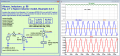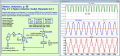# Flyback Converter Magnetic Flux Calculation

#### BlackMelon

Joined Mar 19, 2015
149
Hi

I am designing a flyback converter. The calculation (.jpg file) is based on Daniel W. Hart Power Electronics book. The transformer model consists only of an ideal transformer and its magnetizing inductance (or "primary inductance" in some textbooks).
The datasheet of the transformer's core is the pdf file "pq32_30". The other pdf file is details about the core's material (N87). So, I calculate the magnetic field density (B) at the narrowest area of the core. From this B value (217.032 mT) I do not expect to have any core saturation issue.

However, when I test the real circuit, I can see the peak of the MOSFET's drain current becoming exponential, which means the core saturates.

So, is there something wrong with my calculation?

[EDIT]
I found out why the core saturates. It is because too much flux at the center, not each of the two wings.
Hence, B = flux/Ae = (5.5343*10^-5)/(153.8*10^-6) = 360 mT. The core can handle around 390 mT at 100 C.
So the current 1.4758A is starting point of the saturation. More current = More saturation

#### Attachments

• 324.3 KB Views: 15
• 358.7 KB Views: 5
• 402.3 KB Views: 4
Last edited:

#### crutschow

Joined Mar 14, 2008
32,026
What is the saturation current you calculated?

#### Papabravo

Joined Feb 24, 2006
20,122
Hi

I am designing a flyback converter. The calculation (.jpg file) is based on Daniel W. Hart Power Electronics book. The transformer model consists only of an ideal transformer and its magnetizing inductance (or "primary inductance" in some textbooks).
The datasheet of the transformer's core is the pdf file "pq32_30". The other pdf file is details about the core's material (N87). So, I calculate the magnetic field density (B) at the narrowest area of the core. From this B value (217.032 mT) I do not expect to have any core saturation issue.

However, when I test the real circuit, I can see the peak of the MOSFET's drain current becoming exponential, which means the core saturates.

So, is there something wrong with my calculation?
The effects of saturation do not show up until somewhat higher saturation levels as the following simulations demonstrate:the design value of the inductance is supposed to be 500 μH and withR1 = 100 Ω, the inductance is pretty close to that. The following simulation shows the effect of increased current in the inductor.Notice in the upper trace how the inductance, represented by V(L_value) changes during the cycle of the AC waveform. Notice the definitely non-sinusoidal shape of the current waveform in R1. This is a noticeable saturation effect.

https://www.amazon.com/s?k=Alonso,+...x=alonso,+m.,+inductor,aps,398&ref=nb_sb_noss

#### BlackMelon

Joined Mar 19, 2015
149
What is the saturation current you calculated?
Do you mean the maximum current through the magnetizing inductance? If so, it is 1.4758A.

Papabravo,
Actually, the core is PQ32/30, not EFD25. The material N87 is correct. The gap length is unknown, but it is made such that the magnetizing inductance is 1.5 mH.
Anyway, thank you very much for the information. I will try the simulation using LTSpice like you.

#### Papabravo

Joined Feb 24, 2006
20,122
Do you mean the maximum current through the magnetizing inductance? If so, it is 1.4758A.

Papabravo,
Actually, the core is PQ32/30, not EFD25. The material N87 is correct. The gap length is unknown, but it is made such that the magnetizing inductance is 1.5 mH.
Anyway, thank you very much for the information. I will try the simulation using LTSpice like you.
I understand the differences. I was trying to suggest that your level for imagining saturation to be a problem is too low.

•BlackMelon